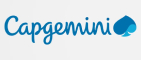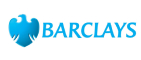New update is available. Click here to update.

# Sum of Two Elements Equals the Third.

Easy0/40
10 mins
90 %20 upvotes+1 more

## Problem Statement

#### An array is said to have a valid triplet {arr[i], arr[j], arr[k]} if there exists three indices i, j and k such that i != j, j != k and i != j and arr[i] + arr[j] = arr[k] or arr[i] + arr[k] = arr[j] or arr[k] + arr[j] = arr[i].

##### For Example:
``````Arr = 10, 5, 5, 6, 2,
In this array, the triplet {10, 5, 5} is valid triplet because, 5 + 5 = 10.
``````

#### Note:

``````The elements in the array need not be distinct.
``````
Detailed explanation ( Input/output format, Notes, Images )
##### Constraints:
``````1 <= T <=  50
1 <= N <= 10^3
1 <= Arr[i] <= 10^4

Time Limit: 1 sec
``````
##### Sample Input 1:
``````2
4
1 1 1 1
5
10 5 5 6 2
``````
##### Sample Output 1:
``````false
true
``````
##### Explanation For Sample Input 1:
``````In the first case, no valid triplet can be formed.

5 5 10 is the triplet in which the sum of two elements {5,5} is equal to the third {10}.
``````
##### Sample Input 2:
``````2
6
1 2 3 1 2 3
6
1 1 2 2 1 1
``````
##### Sample Output 2:
`````` true
true
``````AutocompleteConsole